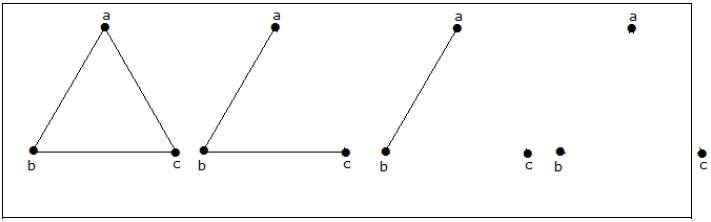# Finding the simple non-isomorphic graphs with n vertices in a graph

MathematicsComputer EngineeringMCA

#### Mathematics for Data Science and Machine Learning using R

64 Lectures 10.5 hours

#### Engineering Mathematics - Numerical Analysis & more

6 Lectures 1 hours

#### Advanced Mathematics Preparation for JEE/CET/CAT

30 Lectures 3.5 hours

## Problem Statement

How many simple non-isomorphic graphs are possible with 3 vertices?

## Solution

There are 4 non-isomorphic graphs possible with 3 vertices. They are shown below.